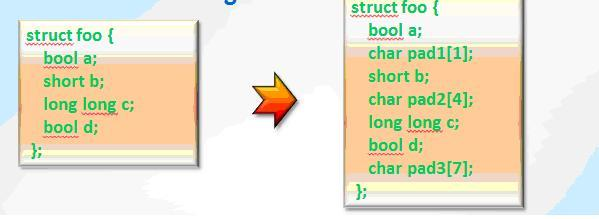Опубликован: 12.07.2012 | Доступ: свободный | Студентов: 338 / 21 | Оценка: 4.00 / 4.20 | Длительность: 11:07:00
Специальности: Программист
Лекция 5:

# Optimizing compiler. Vectorization

< Лекция 4 || Лекция 5: 12345 || Лекция 6 >

### Data alignment

Information about the alignment can be obtained with intrinsic __alignof__. The size and the default alignment of the variable of a type may depend on the compiler. (ia32 or intel64)

``` printf("int:  sizeof=%d align=%d\n",sizeof(a),__alignof__(a));
```

Alignment for ia32 Intel C++ compiler:

```bool           sizeof = 1 alignof = 1
wchar_t        sizeof = 2 alignof = 2
short int      sizeof = 2 alignof = 2
int            sizeof = 4 alignof = 4
long int       sizeof = 4 alignof = 4
long long int  sizeof = 8 alignof = 8
float          sizeof = 4 alignof = 4
double         sizeof = 8 alignof = 8
long double    sizeof = 8 alignof = 8
void*          sizeof = 4 alignof = 4
```

The same rules are used for array alignment.

There is the possibility to force the compiler to align object in a certain way:

```__declspec(align(16)) float x[N];
```Рис. 5.8. Data Structure Alignment

The order of fields in the structure affects the size of the object of a derived type. To reduce the size of the object structure fields should be sorted by descending of its size. You can use __declspec to align structure fields.

```typedef struct aStuct{
__declspec(align(16)) float x[N];
__declspec(align(16)) float y[N];
__declspec(align(16)) float z[N];
};
```Рис. 5.9. The approximate scheme of the loop vectorization

Loop vectorization usually produces three loops: loop for non-aligned staring elements, vectorized loop and tail. Vectorization of loop with small number of iterations can be unprofitable.

### Additional vectorization example

Vector.c

```void Calculate(float * a,float * b,
float * c , int n) {
int i;
for(i=0;i<n;i++) {
a[i] = a[i]+b[i]+c[i];
}
return;
}
```

First argument alignment differs

Main.c

```#include <stdio.h>
#define N 1000
extern void Calculate(float *,float *, float *,int);
int main() {
float x[N],y[N],z[N];
int i,rep;
for(i=0;i<N;i++) {
x[i] = 1;y[i] = 0; z[i] = 1;
}
for(rep=0;rep<10000000;rep++) {
Calculate(&x,&y,&z,N-1);
}
printf("x=%f\n",x);
}

icl  main.c vec.c -O1 –FeA
time a.exe 12.6 s.
```
1. Compiler makes auto vectorization for –O2 or –O3.
```Option -Qvec_report informs about vectorized loops.
icl  main.c vec.c –O2 –Qvec_report –Feb
vec.c(3): (col. 3) remark: LOOP WAS VECTORIZED.
time b.exe         3.67 s.
```

Vectorization is possible because the compiler inserts run-time check for vectorizing when some of the pointers may be not aliased. The application size is enlarged.

2. ```void Calculate(float * resrtict a,float * restrict b, float * restrict c , int n) {
```

To restrict align attribute we need to add option –Qstd=c99

```icl  main.c vec.c –Qstd=c99 –O2 –Qvec_report –Fec
vec.c(3): (col. 3) remark: LOOP WAS VECTORIZED.
time c.exe         3.55 s.
```

Small improvement because of avoiding run-time check

Useful fact: For modern calculation systems performance of aligned and unaligned instructions almost the same when applied to aligned objects.

3. ```int main() {
__declspec(align(16)) float x[N];
__declspec(align(16)) float y[N];
__declspec(align(16)) float z[N];

Calculate(&x,&y,&z,N-1);

void Calculate (float * resrtict a,float * restrict b, float * restrict c , int n) {
Int n;
__assume_aligned(a,16);
__assume_aligned(b,16);
__assume_aligned(c,16);

icl  main.c vec.c –Qstd=c99 –O2 –Qvec_report –Fed
vec.c(3): (col. 3) remark: LOOP WAS VECTORIZED.
time d.exe         3.20 s.
```

This update demonstrates improvement because of the better alignment of vectorized objects. Arrays in main are aligned to 16. With this update all argument pointers are well aligned and the compiler is informed by __assume_aligned directive. It allows to remove the first scalar loop.

### Data alignment

Good array data alignment for SSE: 16B for AVX: 32B

• Data alignment directives:
• C/C++
• Windows: __declspec(align(16)) float X[N];
• Linux/MacOS: float X[N] __attribute__ ((aligned (16));
• Fortran !DIR\$ ATTRIBUTES ALIGN: 16:: A
• Aligned malloc
• _aligned_malloc()
• _mm_malloc()
• Data alignment assertion (16B example)
• C/C++: __assume_aligned(p,16);
• Fortran: !DIR\$ ASSUME_ALIGNED A(1):16
• Aligned loop assertion
• C/C++: #pragma vector aligned
• Fortran: !DIR\$ VECTOR ALIGNED

### Non-unit stride and unit stride access

Well aligned data is better for vectorization because in this case vector register is filled by the single operation. In case with non-unit stride access to array, register filling is more complicated task and vectorization is less profitable.

Auto vectorizer cooperates with loop optimizations for improving access to objects.

There are compiler directives which recommend to make vectorization in case when compiler doesn’t make it because it looks unprofitable.

C/C++

```#pragma vector{aligned|unaligned|always}
#pragma novector
```

Fortran

```!DEC\$ VECTOR ALWAYS
!DEC\$ NOVECTOR
```

### Vectorization of outer loop

Usually auto vectorizer processes the nested loop. Vectorization of the outer loop can be done using "simd" directive.

```#define  N 200
#include<stdio.h>

int main() {
int A[N][N],B[N][N],C[N][N];
int i,j,rep;

for(i=0;i<N;i++)
for(j=0;j<N;j++) {
A[i][j]=i+j;
B[i][j]=2*j-i;
C[i][j]=0;
}
```
```for(rep=0;rep<10000000;rep++) {
#pragma simd
for(i=0;i<N;i++) {
j=0;
while(A[i][j]<=B[i][j] && j<N) {
C[i][j]=C[i][j]+B[j][i]-A[j][i];
j++;
}
}
}
printf("%d\n"C);
}
```
```icl   vec.c -O3 -Qvec- -Fea    (Qvec- disable vectorization)    20.7 s
icl   vec.c -O3 -Qvec_report -Feb                                 17.s
vec.c(17): (col. 3) remark: SIMD LOOP WAS VECTORIZED.
```

< Лекция 4 || Лекция 5: 12345 || Лекция 6 >

### Студенты

Еленеа Бобко
 Беларусь, Минск
Dunduk Dunduk
 Россия